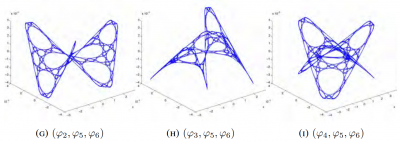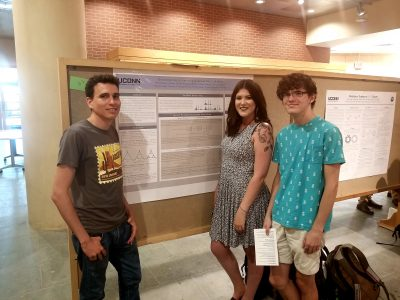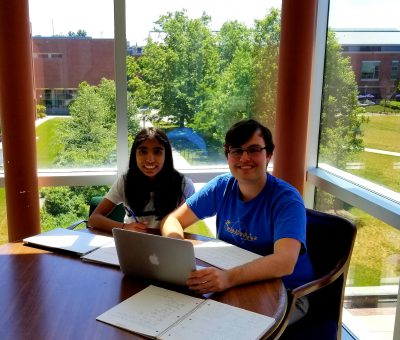# Laplacian eigenmaps 2023REU participants:
Bobita Atkins, Massachusetts College of Liberal Arts
Ashka Dalal, Rose-Hulman Institute of Technology
Natalie Dinin, California State University, Chico
Jonathan Kerby-White, Indiana University Bloomington
Tess McGuinness, University of Connecticut
Tonya Patricks, University of Central Florida
Genevieve Romanelli, Tufts University
Yiheng Su, Colby College

Mentors: Bernard Akwei, Rachel Bailey, Luke Rogers, Alexander Teplyaev

Convergence, optimization and stabilization of singular eigenmaps

B.Akwei, B.Atkins, R.Bailey, A.Dalal, N.Dinin, J.Kerby-White, T.McGuinness, T.Patricks, L.Rogers, G.Romanelli, Y.Su, A.Teplyaev

Eigenmaps are important in analysis, geometry and machine learning, especially in nonlinear dimension reduction.

Versions of the Laplacian eigenmaps of Belkin and Niyogi are a widely used nonlinear dimension reduction technique in data analysis.  Data points in a high dimensional space $$\mathbb{R}^N$$ are treated as vertices of a graph, for example by taking edges between points separated by distance at most a threshold $$\epsilon$$ or by joining each vertex to its $$k$$ nearest neighbors.  A small number $$D$$ of eigenfunctions of the graph Laplacian are then taken as coordinates for the data, defining an eigenmap to $$\mathbb{R}^D$$. This method was motivated by an intuitive argument suggesting that if the original data consisted of $$n$$ sufficiently well-distributed points on a nice manifold $$M$$ then the eigenmap would preserve geometric features of $$M$$.

Several authors have developed rigorous results on the geometric properties of eigenmaps, using a number of different assumptions on the manner in which the points are distributed, as well as hypotheses involving, for example, the smoothness of the manifold and bounds on its curvature.  Typically, they use the idea that under smoothness and curvature assumptions one can approximate the Laplace-Beltrami operator of $$M$$ by an operator giving the difference of the function value and its average over balls of a sufficiently small size $$\epsilon$$, and that this difference operator can be approximated by graph Laplacian operators provided that the $$n$$ points are sufficiently well distributed.

In the present work we consider several model situations where eigen-coordinates can be computed analytically as well as numerically, including the intervals with uniform and weighted measures, square, torus, sphere, and the Sierpinski gasket.  On these examples we investigate the connections between eigenmaps and orthogonal polynomials, how to determine the optimal value of $$\epsilon$$ for a given $$n$$ and prescribed point distribution, and the dependence and stability of the method when the choice of Laplacian is varied.  These examples are intended to serve as model cases for later research on the corresponding problems for eigenmaps on weighted Riemannian manifolds, possibly with boundary, and on some metric measure spaces, including fractals.

Approximation of the eigenmaps of a Laplace operator depends crucially on the scaling parameter $$\epsilon$$. If $$\epsilon$$ is too small or too large, then the approximation is inaccurate or completely breaks down. However, an analytic expression for the optimal $$\epsilon$$ is out of reach. In our work, we use some explicitly solvable models and Monte Carlo simulations to find the approximately optimal value of $$\epsilon$$ that gives, on average, the most accurate approximation of the eigenmaps.

Our study is primarily inspired by the work of Belkin and Niyogi   “Towards a theoretical foundation for Laplacian-based manifold methods.”

Results are presented at the 2023 Young Mathematicians Conference (YMC) at the Ohio State University, a premier annual conference for undergraduate research in mathematics, and at the 2024 Joint Mathematics Meetings (JMM) in San Francisco, the largest mathematics gathering in the world.

# Geodesic interpolation on Sierpiński gaskets

• ### Laura A. LeGare

University of Notre Dame, USA

• ### Cory W. McCartan

Harvard University, Cambridge, USA

• ### Luke G. Rogers

University of Connecticut, Storrs, USA

• DOI 10.4171/JFG/100
• JFG VOL. 8, NO. 2 PP. 117–152
• 2018 project page

# Resistance scaling on affine carpets

## Overview

A celebrated result in analysis and probability on fractals is the construction of a diffusion on the standard Sierpinski Carpet by Barlow and Bass. One key part of their argument is a pair of upper and lower estimates for the resistances of precarpets: if $$K_n$$ denotes the level $$n$$ approximation of the carpet and $$E_n$$ is the minimal Dirichlet energy of a function that is identically 1 on one side of the carpet and identically 0 on the other side, then there are constants $$0<c\leq C< \infty$$ so that $$c\rho^n\leq E_n\leq C\rho^n$$. Estimates for $$\rho$$ are known but the exact value is not.

The Sierpinski-type carpets to which the preceding estimates of resistance have been extended are all self-similar. By contrast, in the setting of post-critically finite fractals, resistance scaling has been successfully studied also in the self-affine case, initially by Fitzsimmons, Hambly and Kumagai.

The goal of this project was to investigate what aspects of the Barlow-Bass approach to resistance estimation on carpets could be extended to the self-affine case, and to make numerical computations of the behavior of resistance in this setting and its dependence on the affine scalings.

# Decimation structure of the spectra of self-similar groups

## Group Members## Overview

Physicists and mathematicians have used the self-similar nature of certain fractals to develop and study analytical structures on fractal spaces. We examine the analytical structure of a class of fractals that arise as limit sets of the Schreier graphs of the action of self-similar groups on infinite n-ary trees. In particular, we consider how the spectrum of a Laplacian operator on one level of a Schreier graph relates to the spectrum on the next level, a technique known as spectral decimation.

Grigorchuk and collaborators have developed a method to spectrally decimate Schreier graphs of several
important self-similar groups, and have derived significant consequences about the structure of amenable groups. Their method is related to a notion of spectral similarity arising from the work of Fukushima-Shima and Malozemov-Teplyaev. In the latter, a sufficient condition for spectral decimation for fractal graphs is obtained. We consider the analogous question for Schreier graphs of self-similar groups with the goal of understanding the class to which Grigorchuk’s approach is applicable.

# Can we hear the shape of a fractal? Spectral analysis of self-similar sets

## Group Members## Overview

Analytic structures on fractals have been analyzed extensively in the past 50 years both because of their interesting mathematical properties and their potential applications in physics. One important question in this area is how the spectrum of a Laplacian on a fractal reflects its geometry; one version of the corresponding problem for domains in Euclidean space was famously described in Kac’s question ”Can you hear the shape of a drum?”.
Some features of the spectra of self-similar sets, such as the asymptotic behavior of the eigenvalue counting function, can be obtained using renewal theory (as in the work of Kigami-Lapidus), but our interest is in more precise results that give the locations and multiplicities of eigenvalues explicitly. These are connected to a long strand of research in mathematical physics about the structure of spectra of Schrodinger operators ¨ and their relation to topological invariants of the underlying space (prominent results in this area are due to Landau, Peierls, Harper, Moser, Bellissard, and, recently, Avila and Jitomirskaya). One name for these results is gap-labeling theorems. For certain highly-symmetric self-similar sets, the computation of the gap structure of the Laplacian spectrum is possible using spectral decimation. We use this method to explicitly compute the gap structure for the Laplacian on a particular two-point self-similar graph and its fractal limit, and for Sierpinski graphs and the Sierpinski gasket.

# Resistance scaling on the Octacarpet

## Group Members## Overview

The study of analytic structures on self-similar fractal sets was initiated by physicists who discovered that heat flow on such sets had sub-Gaussian rather than Gaussian scaling, indicating that the fundamental physics of these sets was very different than on manifolds. These results were first made rigorous for sets with a finite ramification property, but in the late 1980s, Barlow and Bass developed a corresponding theory on a class of generalized Sierpinski carpets. Their approach depends on taking a (weak) limit of Brownian motions on a suitable sequence of closed sets that intersect to the carpet. A key step in proving that the limiting object has sub-Gaussian scaling is showing that the resistance of the approximating domain of scale n is bounded above and below by ρ^n
for a factor ρ that depends on the carpet. Computing the exact value of ρ remains an open problem.

We consider the resistance scaling problem for the octacarpet, and more generally for 4N-carpets, with
the goal of showing analogous bounds for the resistance and obtaining numerical estimates for the resistance scaling factors.

# Geodesic Interpolation on the Sierpinski Gasket

## Overview

Geodesics (shortest paths) on manifolds such as planes and spheres are well understood.  Geodesics on fractal sets such as the Sierpinski Triangle are much more complicated.  We begin by constructing algorithms for building shortest paths and provide explicit formulas for computing their lengths.  We then turn to the question of interpolation along geodesics—given two subsets of the Sierpinski Triangle, we “slide” points in one set along geodesics to the other set.  We construct a measure along the interpolated sets which formalizes a notion of the interpolation of a distribution of mass, and we prove interesting self-similarity relations about this measure.

# Computations on the Koch Snowflake with Boundary and Interior Energies

## Group Members## Overview

At the end of the 20th century studies had been conducted on the Koch Snowflake which had been motivated through work done by physicists on “fractal drum” experiments. These investigations focused on the eigenfunctions of the negative Dirichlet Lapcian generated on a planar domain with a fractal boundary, particularly with the condition that the boundary be set to zero. Here we study the eigenfunctions on the Koch Snowflake with a non-zero boundary condition and we consider a Laplacian defined on the boundary. To generate an n-level fractal and apply the Laplacian matrix, Python programming was implemented for both the n-level fractal and Laplacian matrix. This then gives us insight into the eigenvalues and visualization of the corresponding eigenfunctions on the Koch Snowflake. Initial observations indicate a kind of localization of the eigenfunctions on the fractal boundary.

# Lipschitz Continuity of Laplacian Eigenfunctions on a class of postcritically finite (PCF) self-similar sets

## Group Members## Overview

We prove a general result for Lipschitz and Hölder continuity of functions defined on a class of postcritically finite (PCF) self-similar sets. Intuition for this theorem comes from formulating arguments on the unit interval, which is well understood in a classical setting. We generalize these results for many kinds of self-similar sets endowed with different measures and metrics. As a corollary to this general result, we prove that eigenfunctions of the Laplacian on the harmonic Sierpinski Gasket are Lipschitz continuous.

# Probability, Analysis and Mathematical Physics on Fractals 2018

Each year we are looking for a group of undergraduate students to work on Probability, Analysis and Mathematical Physics on Fractals. The aim of the projects will be exploration of differential equations and various operators on fractal domains. Students in the project are supposed to have the usual background in linear algebra and differential equations. Knowledge of Matlab, Mathematica, other computer algebra systems, or programming, as well as proof writing, mathematical analysis, and probability may be helpful but is not required. Previous undergraduate work includes published papers on the eigenmodes (vibration modes) of the Laplacian (2nd derivative) of functions that live on Sierpinski gasket type fractals, and the electrical resistance of fractal networks, as well as work on Laplacians on projective limit spaces. The exact choice of the topics to study will depend on the students’ background and interests. Besides being interesting, taking part in a research project like this may be very useful in the future (for instance, when applying to graduate schools).